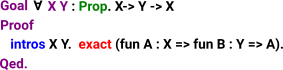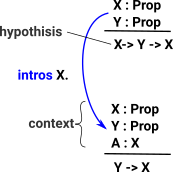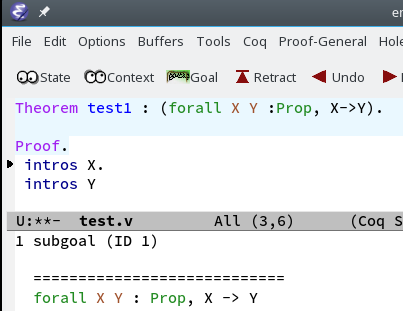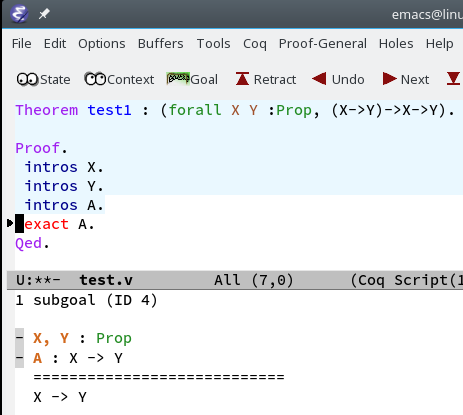# Coq

## Install Coq

Instructions available here:

https://github.com/coq/coq/wiki/Installation-of-Coq-on-Linux

 Coq is not available directly on openSUSE so I installed from open build service.installs in:

• //usr/lib64/coq
• //usr/lib64/ocaml

## Install Proof-General

Assuming Emacs with melpa is already installed (if not see this page ) install proof-general into Emacs:

#### Install proof-general

M-x package-refresh-contents

M-x package-install <cr>
proof-general## Using Coq

Gallina is the specification language of Coq:

https://coq.inria.fr/refman/language/gallina-specification-language.html

It is important that this language cannot prove false. Because of this the language is not turing complete, it always terminates (has weak normalization property).

#### Types in Gallina

:Type - hierarchy of types

:Prop - propositions are outside this hierarchy

Gallina is a programming language with dependant and inductive types. Every function is total.

#### Examples

 data: ```Inductive day : Type := | monday : day | tuesday : day | wednesday : day | thursday : day | friday : day | saturday : day | sunday : day.``` functions: ```Definition next_weekday (d:day) : day := match d with | monday => tuesday | tuesday => wednesday | wednesday => thursday | thursday => friday | friday => monday | saturday => monday | sunday => monday end.```

## Examples

Function (lambda) examples.

 The tools such as proof-general allow proofs to be derived interactivly. Each tactic used changes some internal proof state. So we are, sort of, seralising the proof.Instead of specifying A & B we could specify lambda.### intros

Takes hypothisis and puts into context,

Use when we have implication or forall.

Constucts a lambdaAt the start of the proof the context is empty and the assumptions are part of the conclusion.When we step to

intros X

this moves X:Prop to the context.## Proofs with Quantifiers## Syntax

 Check let Eval x in Inductive x := Define a type (data) x by giving its constructor. Definition x := Define a constant, could be a function Require Import Load a library, for instance: Bool Arith List Open Scope Print Locate Fixpoint Define a recursive function. Notation "( x , y )" := (pair x y). Allows us to define an alternative notation. Example, Theorem, Lemma, Fact and Remark These keywords mean the same thing. ```Proof ... QED.``` Tactics tell Coq how it should check the correctness of some claim we are making ```Proof ...Admitted.``` give up trying to prove this theorem and just accept it as a given.

#### Tactics:

 reflexivity. both sides of equation are the same simpl. simplify intros H. Move the hypothesis H into the context. Allows us to reason by assuming the hypothesis rewrite -> H. Rewrite the goal using the hypothesis H destruct n as [| n']. n is variable name to be introduced apply apply a function. So if we have (X->Y) and X we can apply to get Y. unfold assert Allows large proofs to be broken into sub-proofs, we can assert and prove in place, rather than creating external top level proofs.

## Comparing Coq to Idris

In order to get some insight into the design of Coq I think it helps to compare it with other programs. So here is a comparison with Idris (see this page), Idris is a more general language but it also does proofs.

Coq Idris
Booleans
```Inductive bool : Type :=
| true : bool
| false : bool.
```
`data Bool = False | True`
not
```Definition negb (b:bool) : bool :=
match b with
| true => false
| false => true
end.```
```not : Bool -> Bool
not True = False
not False = True```
and
```Definition andb (b1:bool) (b2:bool) : bool :=
match b1 with
| true => b2
| false => false
end.```
```(&&) : Bool -> Lazy Bool -> Bool
(&&) True x  = x
(&&) False _ = False```
or
```Definition orb (b1:bool) (b2:bool) : bool :=
match b1 with
| true => true
| false => b2
end.```
```(||) : Bool -> Lazy Bool -> Bool
(||) False x = x
(||) True _ = True```
Numbers
```Inductive nat : Set :=
| O : nat
| S : nat -> nat.```
```data Nat : Type where
Z : Nat
S : Nat -> Nat
```
plus
```Fixpoint plus (n m : nat) : nat :=
match n with
| O => m
| S n' => S (plus n' m)
end.```
```plus : Nat -> Nat -> Nat
plus Z     m = m
plus (S k) m = S (plus k m)```

```Theorem plus_O_n : forall n:nat, 0 + n = n.
Proof.
simpl. reflexivity. Qed.```
```plusReduces : (n:Nat) -> plus Z n = n
plusReduces n = Refl```

`x`
```plusReducesZ : (n:Nat) -> n = plus n Z
plusReducesZ Z = Refl
plusReducesZ (S k) = cong (plusReducesZ k)```

`x`
`x`

`x`
`x`

`x`
`x`

`x`
`x`

`x`
`x`

`x`
`x`

`x`
`x`

## HoTT

Idris and HoTT:

 metadata block see also: Correspondence about this page Book Shop - Further reading. Where I can, I have put links to Amazon for books that are relevant to the subject, click on the appropriate country flag to get more details of the book or to buy it from them.Fast Track UML 2.0 - useful for people who know some UML but are upgrading to 2.0 Commercial Software Shop Where I can, I have put links to Amazon for commercial software, not directly related to the software project, but related to the subject being discussed, click on the appropriate country flag to get more details of the software or to buy it from them.

This site may have errors. Don't use for critical systems.